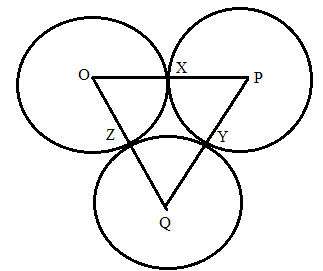Chapter 6.3, Problem 41E### Elementary Geometry for College St...

6th Edition
Daniel C. Alexander + 1 other
ISBN: 9781285195698

#### Solutions

Chapter
Section### Elementary Geometry for College St...

6th Edition
Daniel C. Alexander + 1 other
ISBN: 9781285195698
Textbook Problem
1 views

# Circles O, P, and Q are tangent (as shown for Exercise 40 on page 296) at points X, Y, and Z. being as specific as possible, explain what type of triangle Δ P Q O is if:a) O X = 3 , P Y = 4 , Q Z = 1 b) O X = 2 , P Y = 2 , Q Z = 2 .To determine

a)

To find:

To explain what type of triangle ΔPQO is if:

OX=3,PY=4,QZ=1

Explanation

Given that, circles O, P, and Q are tangent (as shown) at points X, Y, and Z.

Then the value of OX=3,PY=4,QZ=1.

The diagrammatic representation is given below,

To find the measures of the sides of the triangle ΔPQO is given below,

OP=OX+PXPQ=PY+QYOQ=QZ+OZ

We know that OX=OZ,PX=PY,QY=QZ since radius of the circle is same for all points on the circle.

Substitute the value of OX=3,PY=4,QZ=1 and OX=OZ,PX=PY,QY=QZ to get the following,

OP

To determine

b)

To find:

To explain what type of triangle ΔPQO is if:

OX=2,PY=2,QZ=2

### Still sussing out bartleby?

Check out a sample textbook solution.

See a sample solution

#### The Solution to Your Study Problems

Bartleby provides explanations to thousands of textbook problems written by our experts, many with advanced degrees!

Get Started

#### In Problems and , use technology to find the product of the following matrices.

Mathematical Applications for the Management, Life, and Social Sciences

#### Evaluate the expression sin Exercises 116. 2322

Finite Mathematics and Applied Calculus (MindTap Course List)

#### If f(x) = 2x + In x, find f1(2).

Single Variable Calculus: Early Transcendentals, Volume I

#### True or False: .

Study Guide for Stewart's Single Variable Calculus: Early Transcendentals, 8th